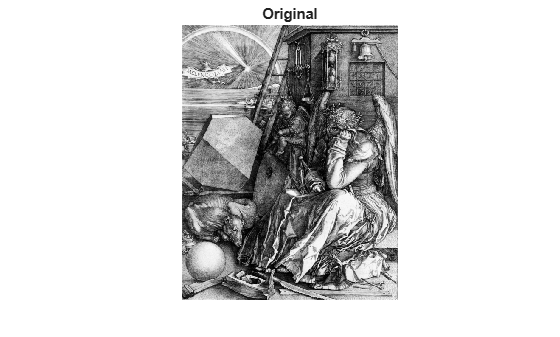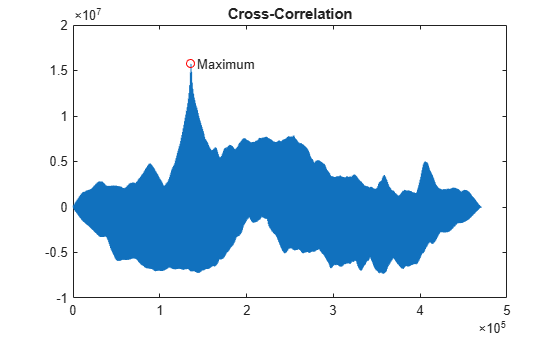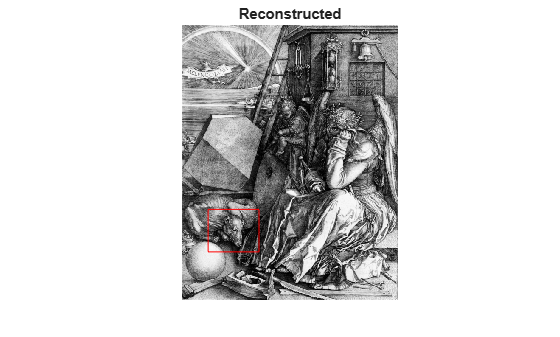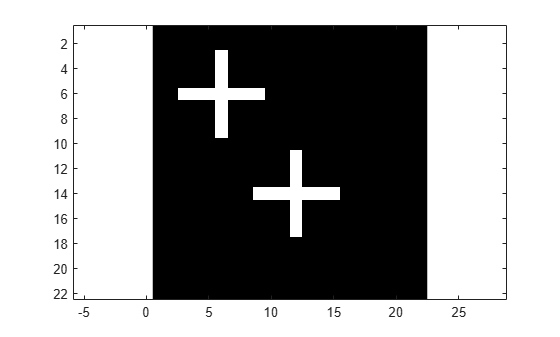Documentation

This is machine translation

Mouseover text to see original. Click the button below to return to the English version of the page.

xcorr2

2-D cross-correlation

Syntax

``c = xcorr2(a,b)``
``c = xcorr2(a)``

Description

example

````c = xcorr2(a,b)` returns the cross-correlation of matrices `a` and `b` with no scaling. `xcorr2` is the two-dimensional version of `xcorr`.```
````c = xcorr2(a)` is the autocorrelation matrix of input matrix `a`. This syntax is equivalent to `xcorr2(a,a)`.```

Examples

collapse all

Create two matrices, `M1` and `M2`.

```M1 = [17 24 1 8 15; 23 5 7 14 16; 4 6 13 20 22; 10 12 19 21 3; 11 18 25 2 9]; M2 = [8 1 6; 3 5 7; 4 9 2];```

`M1` is 5-by-5 and `M2` is 3-by-3, so their cross-correlation has size (5+3-1)-by-(5+3-1), or 7-by-7. In terms of lags, the resulting matrix is

`$C=\left(\begin{array}{ccccccc}{c}_{-2,-2}& {c}_{-2,-1}& {c}_{-2,0}& {c}_{-2,1}& {c}_{-2,2}& {c}_{-2,3}& {c}_{-2,4}\\ {c}_{-1,-2}& {c}_{-1,-1}& {c}_{-1,0}& {c}_{-1,1}& {c}_{-1,2}& {c}_{-1,3}& {c}_{-1,4}\\ {c}_{0,-2}& {c}_{0,-1}& {c}_{0,0}& {c}_{0,1}& {c}_{0,2}& {c}_{0,3}& {c}_{0,4}\\ {c}_{1,-2}& {c}_{1,-1}& {c}_{1,0}& {c}_{1,1}& {c}_{1,2}& {c}_{1,3}& {c}_{1,4}\\ {c}_{2,-2}& {c}_{2,-1}& {c}_{2,0}& {c}_{2,1}& {c}_{2,2}& {c}_{2,3}& {c}_{2,4}\\ {c}_{3,-2}& {c}_{3,-1}& {c}_{3,0}& {c}_{3,1}& {c}_{3,2}& {c}_{3,3}& {c}_{3,4}\\ {c}_{4,-2}& {c}_{4,-1}& {c}_{4,0}& {c}_{4,1}& {c}_{4,2}& {c}_{4,3}& {c}_{4,4}\end{array}\right).$`

As an example, compute the element ${c}_{0,2}$ (or `C(3,5)` in MATLAB®, since `M2` is 3-by-3). Line up the two matrices so their `(1,1)` elements coincide. This placement corresponds to ${c}_{0,0}$. To find ${c}_{0,2}$, slide `M2` two rows to the right.Now `M2` is on top of the matrix `M1(1:3,3:5)`. Compute the element-by-element products and sum them. The answer should be

`$1×8+7×3+13×4+8×1+14×5+20×9+15×6+16×7+22×2=585.$`

```[r2,c2] = size(M2); CC = sum(sum(M1(0+(1:r2),2+(1:c2)).*M2))```
```CC = 585 ```

Verify the result using `xcorr2`.

```D = xcorr2(M1,M2); DD = D(0+r2,2+c2)```
```DD = 585 ```

Given a matrix $\mathcal{X}$ of size $M×N$ and a matrix $\mathcal{H}$ of size $P×Q$, their two-dimensional cross-correlation, $\mathcal{C}=\mathcal{X}\star \mathcal{H}$, is a matrix of size $\left(M+P-1\right)×\left(N+Q-1\right)$ with elements

`$\mathcal{C}\left(k,l\right)=Tr\phantom{\rule{0.16666666666666666em}{0ex}}\left\{\underset{}{\overset{\sim }{\mathcal{X}}}{\underset{}{\overset{\sim }{\mathcal{H}}}}_{kl}^{†}\right\}\phantom{\rule{2em}{0ex}}\begin{array}{c}1\le k\le M+P-1,\\ 1\le l\le N+Q-1.\end{array}$`

$Tr$ is the trace and the dagger denotes Hermitian conjugation. The matrices $\underset{}{\overset{\sim }{\mathcal{X}}}$ and ${\underset{}{\overset{\sim }{\mathcal{H}}}}_{kl}$ have size $\left(M+2\left(P-1\right)\right)×\left(N+2\left(Q-1\right)\right)$ and nonzero elements given by

`$\underset{}{\overset{～}{\mathcal{X}}}\left(m,n\right)=\mathcal{X}\left(m-P+1,n-Q+1\right),\phantom{\rule{1em}{0ex}}\begin{array}{c}P\le m\le M+P-1,\\ Q\le n\le N+Q-1\end{array}$`

and

`${\underset{}{\overset{～}{\mathcal{H}}}}_{kl}\left(p,q\right)=\mathcal{H}\left(p-k+1,q-l+1\right),\phantom{\rule{1em}{0ex}}\begin{array}{c}k\le p\le P+k-1,\\ l\le q\le Q+l-1.\end{array}$`

Calling `xcorr2` is equivalent to this procedure for general complex matrices of arbitrary size.

Create two complex matrices, $\mathcal{X}$ of size $7×22$ and $\mathcal{H}$ of size $6×17$.

```X = randn([7 22])+1j*randn([7 22]); H = randn([6 17])+1j*randn([6 17]); [M,N] = size(X); m = 1:M; n = 1:N; [P,Q] = size(H); p = 1:P; q = 1:Q;```

Initialize $\underset{}{\overset{\sim }{\mathcal{X}}}$ and $\mathcal{C}$.

```Xt = zeros([M+2*(P-1) N+2*(Q-1)]); Xt(m+P-1,n+Q-1) = X; C = zeros([M+P-1 N+Q-1]);```

Compute the elements of $\mathcal{C}$ by looping over $k$ and $l$. Reset ${\underset{}{\overset{\sim }{\mathcal{H}}}}_{kl}$ to zero at each step. Save time and memory by summing element products instead of multiplying and taking the trace.

```for k = 1:M+P-1 for l = 1:N+Q-1 Hkl = zeros([M+2*(P-1) N+2*(Q-1)]); Hkl(p+k-1,q+l-1) = H; C(k,l) = sum(sum(Xt.*conj(Hkl))); end end max(max(abs(C-xcorr2(X,H))))```
```ans = 1.4648e-14 ```

The answer coincides to machine precision with the output of `xcorr2`.

Use cross-correlation to find where a section of an image fits in the whole. Cross-correlation enables you to find the regions in which two signals most resemble each other. For two-dimensional signals, like images, use `xcorr2`.

Load a black-and-white test image into the workspace. Display it with `imagesc`.

```load durer img = X; White = max(max(img)); imagesc(img) axis image off colormap gray title('Original')```Select a rectangular section of the image. Display the larger image with the section missing.

```x = 435; X = 535; szx = x:X; y = 62; Y = 182; szy = y:Y; Sect = img(szx,szy); kimg = img; kimg(szx,szy) = White; kumg = White*ones(size(img)); kumg(szx,szy) = Sect; subplot(1,2,1) imagesc(kimg) axis image off colormap gray title('Image') subplot(1,2,2) imagesc(kumg) axis image off colormap gray title('Section')```Use `xcorr2` to find where the small image fits in the larger image. Subtract the mean value so that there are roughly equal numbers of negative and positive values.

```nimg = img-mean(mean(img)); nSec = nimg(szx,szy); crr = xcorr2(nimg,nSec);```

The maximum of the cross-correlation corresponds to the estimated location of the lower-right corner of the section. Use `ind2sub` to convert the one-dimensional location of the maximum to two-dimensional coordinates.

```[ssr,snd] = max(crr(:)); [ij,ji] = ind2sub(size(crr),snd); figure plot(crr(:)) title('Cross-Correlation') hold on plot(snd,ssr,'or') hold off text(snd*1.05,ssr,'Maximum')```Place the smaller image inside the larger image. Rotate the smaller image to comply with the convention that MATLAB® uses to display images. Draw a rectangle around it.

```img(ij:-1:ij-size(Sect,1)+1,ji:-1:ji-size(Sect,2)+1) = rot90(Sect,2); imagesc(img) axis image off colormap gray title('Reconstructed') hold on plot([y y Y Y y],[x X X x x],'r') hold off```Shift a template by a known amount and recover the shift using cross-correlation.

Create a template in an 11-by-11 matrix. Create a 22-by-22 matrix and shift the original template by 8 along the row dimension and 6 along the column dimension.

```template = 0.2*ones(11); template(6,3:9) = 0.6; template(3:9,6) = 0.6; offsetTemplate = 0.2*ones(22); offset = [8 6]; offsetTemplate((1:size(template,1))+offset(1), ... (1:size(template,2))+offset(2)) = template;```

Plot the original and shifted templates.

```imagesc(offsetTemplate) colormap gray hold on imagesc(template) axis equal```Cross-correlate the two matrices and find the maximum absolute value of the cross-correlation. Use the position of the maximum absolute value to determine the shift in the template. Check the result against the known shift.

```cc = xcorr2(offsetTemplate,template); [max_cc, imax] = max(abs(cc(:))); [ypeak, xpeak] = ind2sub(size(cc),imax(1)); corr_offset = [(ypeak-size(template,1)) (xpeak-size(template,2))]; isequal(corr_offset,offset)```
```ans = logical 1 ```

The shift obtained from the cross-correlation equals the known template shift in the row and column dimensions.

This example requires Parallel Computing Toolbox™ software. Refer to GPU Support by Release (Parallel Computing Toolbox) to see what GPUs are supported.

Shift a template by a known amount and recover the shift using cross-correlation.

Create a template in an 11-by-11 matrix. Create a 22-by-22 matrix and shift the original template by 8 along the row dimension and 6 along the column dimension.

```template = 0.2*ones(11); template(6,3:9) = 0.6; template(3:9,6) = 0.6; offsetTemplate = 0.2*ones(22); offset = [8 6]; offsetTemplate((1:size(template,1))+offset(1), ... (1:size(template,2))+offset(2)) = template;```

Put the original and shifted template matrices on your GPU using `gpuArray` objects.

```template = gpuArray(template); offsetTemplate = gpuArray(offsetTemplate);```

Compute the cross-correlation on the GPU.

`cc = xcorr2(offsetTemplate,template);`

Return the result to the MATLAB® workspace using `gather`. Use the maximum absolute value of the cross-correlation to determine the shift, and compare the result with the known shift.

```cc = gather(cc); [max_cc,imax] = max(abs(cc(:))); [ypeak,xpeak] = ind2sub(size(cc),imax(1)); corr_offset = [(ypeak-size(template,1)) (xpeak-size(template,2))]; isequal(corr_offset,offset)```
```ans = logical 1 ```

Input Arguments

collapse all

Input arrays, specified as matrices or `gpuArray` objects.

See Run MATLAB Functions on a GPU (Parallel Computing Toolbox) and GPU Support by Release (Parallel Computing Toolbox) for details on using `xcorr2` with `gpuArray` objects.

Example: `sin(2*pi*(0:9)'/10)*sin(2*pi*(0:13)/20)` specifies a two-dimensional sinusoidal surface.

Example: `gpuArray(sin(2*pi*(0:9)'/10)*sin(2*pi*(0:13)/20))` specifies a two-dimensional sinusoidal surface as a `gpuArray` object.

Data Types: `single` | `double`
Complex Number Support: Yes

Output Arguments

collapse all

2-D cross-correlation or autocorrelation matrix, returned as a matrix or a `gpuArray` object.

collapse all

2-D Cross-Correlation

The 2-D cross-correlation of an M-by-N matrix, X, and a P-by-Q matrix, H, is a matrix, C, of size M+P–1 by N+Q–1. Its elements are given by

`$C\left(k,l\right)=\sum _{m=0}^{M-1}\sum _{n=0}^{N-1}X\left(m,n\right)\text{\hspace{0.17em}}\overline{H}\left(m-k,n-l\right),\text{ }\text{ }\text{ }\begin{array}{c}-\left(P-1\right)\le k\le M-1,\\ -\left(Q-1\right)\le l\le N-1,\end{array}$`

where the bar over H denotes complex conjugation.

The output matrix, C(k,l), has negative and positive row and column indices.

• A negative row index corresponds to an upward shift of the rows of H.

• A negative column index corresponds to a leftward shift of the columns of H.

• A positive row index corresponds to a downward shift of the rows of H.

• A positive column index corresponds to a rightward shift of the columns of H.

To cast the indices in MATLAB® form, add the size of H: the element C(k,l) corresponds to `C(k+P,l+Q)` in the workspace.

For example, consider this 2-D cross-correlation:

```X = ones(2,3); H = [1 2; 3 4; 5 6]; % H is 3 by 2 C = xcorr2(X,H)```
```C = 6 11 11 5 10 18 18 8 6 10 10 4 2 3 3 1```

The `C(1,1)` element in the output corresponds to C(1–3,1–2) = C(–2,–1) in the defining equation, which uses zero-based indexing. To compute the `C(1,1)` element, shift `H` two rows up and one column to the left. Accordingly, the only product in the cross-correlation sum is `X(1,1)*H(3,2) = 6`. Using the defining equation, you obtain

`$C\left(-2,-1\right)=\sum _{m=0}^{1}\sum _{n=0}^{2}X\left(m,n\right)\text{\hspace{0.17em}}\overline{H}\left(m+2,n+1\right)=X\left(0,0\right)\text{\hspace{0.17em}}\overline{H}\left(2,1\right)=1\text{\hspace{0.17em}}×\text{\hspace{0.17em}}6=6,$`

with all other terms in the double sum equal to zero.